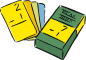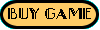### The Numbers Minus GameThe Numbers Minus game introduces the child to the idea of subtraction.

The child selects two numbers to subtract and the total number of objects remaining is counted out. Negative numbers are introduced.

Let your child have fun learning subtraction.

The Numbers Minus Game is intended for age 4 through 7.
The Numbers Minus Game is rated B L, V I, C B, D F, M I, N I
FREE